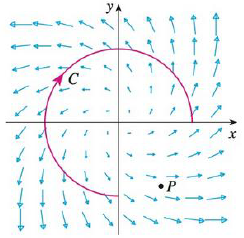Chapter 16, Problem 1RE

Chapter
Section
Textbook Problem

A vector field F, a curve C, and a point P are shown.(a) Is ∫C F · dr positive, negative, or zero? Explain.(b) Is div F(P) positive, negative, or zero? Explain.(a)

To determine

To explain: CFdr is positive, negative or zero.

Explanation

Given data:

The figure of vector field F, a curve C, and a point P is given.

In given figure, the vector starting on point C directed in opposite direction to C, so the tangential component FT is negative.

Represent dr in terms of Tds .

dr=Tds

Find the value of CFdr .

CFdr=C

(b)

To determine

To explain: divF(P) is positive, negative or zero.

Still sussing out bartleby?

Check out a sample textbook solution.

See a sample solution

The Solution to Your Study Problems

Bartleby provides explanations to thousands of textbook problems written by our experts, many with advanced degrees!

Get Started

In Exercise 11-14, factor the expression. y23x+94

Calculus: An Applied Approach (MindTap Course List)

Convert the expressions in Exercises 31-36 to positive exponent form. 10.3x265x1

Finite Mathematics and Applied Calculus (MindTap Course List)

In Exercises 4562, find the values of x that satisfy the inequality (inequalities). 51. x + 1 4 or x + 2 1

Applied Calculus for the Managerial, Life, and Social Sciences: A Brief Approach

The curvature of at t = 0 is:

Study Guide for Stewart's Multivariable Calculus, 8th

True or False: The graph at the right is the graph of an even function.

Study Guide for Stewart's Single Variable Calculus: Early Transcendentals, 8th